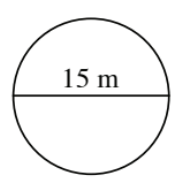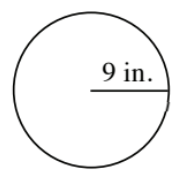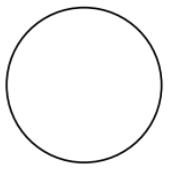### Home > CC2 > Chapter 9 > Lesson 9.1.2 > Problem9-32

9-32.

Find the area and circumference of the circles in parts (a) and (b) and the radius in part (c). You may want to refer to the Math Notes box in this lesson.

1.$\text{Circumference}=\pi\left(\text{diameter}\right)$
$\text{Area}=\left(\text{radius}\right)^2\pi$

What measurement is given in the diagram?

To find the circumference, substitute the diameter into the equation.To find the area, you must find the radius from the diameter.

Remember that the diameter is $2$ times the radius.

$\text{C}=\pi(15)$
$\text{A}=(7.5)^2\pi$

$\text{A}=176.7$ m2
$\text{C}=47.1$ m

1.See part (a).

1. $C=314 \text{ cm}$In this equation, you are given the circumference. With this, you must find the radius in order to find the area.

Since $\text{Circumference}=2\left(\text{radius}\right)\pi$

$\text{Radius }=\frac{\text{Circumference}}{2\pi}$